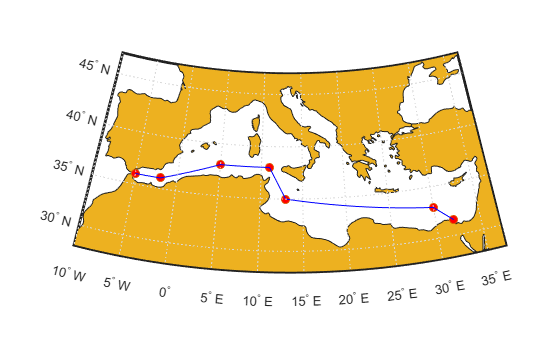# track

Geographic track points from waypoints

## Syntax

``[lattrk,lontrk] = track(waypts)``
``[lattrk,lontrk] = track(lat,lon)``
``[lattrk,lontrk] = track(___,units)``
``[lattrk,lontrk] = track(lat,lon,ellipsoid)``
``[lattrk,lontrk] = track(lat,lon,ellipsoid,units,npts)``
``[lattrk,lontrk] = track(method,___)``
``mat = track(___)``

## Description

### Track Points Along Unit Sphere

````[lattrk,lontrk] = track(waypts)` finds the latitude and longitude coordinates of track points between the waypoints specified by `waypts`. This syntax references the coordinates to a unit sphere and calculates track points using rhumb lines.```

example

````[lattrk,lontrk] = track(lat,lon)` finds the coordinates of track points between the waypoints with coordinates `lat` and `lon`. This syntax is equivalent to `track(waypts)`, where `waypts = [lat lon]`.```
````[lattrk,lontrk] = track(___,units)` specifies the angle units `units` for the inputs and outputs.```

### Track Points Along Ellipsoid

example

````[lattrk,lontrk] = track(lat,lon,ellipsoid)` specifies a reference ellipsoid for the coordinates.```
````[lattrk,lontrk] = track(lat,lon,ellipsoid,units,npts)` specifies the number of points to calculate between each waypoint.```

````[lattrk,lontrk] = track(method,___)`, where `method` is `"gc"`, finds track points along great circles (for a sphere) or geodesics (for an ellipsoid). The default for `method` is `"rh"`, which finds track points using rhumb lines.```
````mat = track(___)` returns the latitude and longitude coordinates of the track points in the matrix `mat`.```

## Examples

collapse all

Specify the coordinates of several waypoints in the Mediterranean Sea. Then, find the coordinates of track points between the waypoints.

```lat = [36 36 38 38 35 33 31.5]; lon = [-5 -2 5 11 13 30 32]; [lattrk,lontrk] = track(lat,lon);```

Create a map of the area. Display the waypoints using red circle markers and the track points using a blue line.

```GT = readgeotable("landareas.shp"); worldmap([28 47],[-10 37]) geoshow(GT) geoshow(lat,lon,"DisplayType","point","Marker","o","MarkerFaceColor","r") geoshow(lattrk,lontrk,"DisplayType","line","Color","b")```Import a shapefile containing worldwide land areas into the workspace as a geospatial table. Extract the reference ellipsoid for the land areas from the table.

```GT = readgeotable("landareas.shp"); ellipsoid = GT.Shape.GeographicCRS.Spheroid;```

Specify the coordinates of several waypoints in an area surrounding the Mediterranean Sea. Then, reference the coordinates to the ellipsoid and find the coordinates of track points between the waypoints.

```lat = [36 36 38 38 35 33 31.5]; lon = [-5 -2 5 11 13 30 32]; [lattrk,lontrk] = track(lat,lon,ellipsoid);```

Create a map of the area. Display the waypoints using red circle markers and the track points using a blue line.

```worldmap([28 47],[-10 37]) geoshow(GT) geoshow(lat,lon,"DisplayType","point","Marker","o","MarkerFaceColor","r") geoshow(lattrk,lontrk,"DisplayType","line","Color","b")```## Input Arguments

collapse all

Latitude and longitude coordinates of the waypoints, specified as a two-column matrix. The first column of the matrix contains the latitude coordinates and the second column of the matrix contains the longitude coordinates.

Data Types: `single` | `double`

Latitude coordinates of the waypoints, specified as a vector.

The size of `lat` must match the size of `lon`.

Data Types: `single` | `double`

Longitude coordinates of the waypoints, specified as a vector.

The size of `lat` must match the size of `lon`.

Data Types: `single` | `double`

Angle unit, specified as one of these options:

• `"degrees"` — Degrees

• `"radians"` — Radians

Data Types: `char` | `string`

Reference ellipsoid, specified as a `referenceSphere` object, a `referenceEllipsoid` object, an `oblateSpheroid` object, or a two-element vector of the form `[semimajor_axis eccentricity]`, where `semimajor_axis` is the length of the semimajor axis and `eccentricity` is the eccentricity. The values `semimajor_axis` and `eccentricity` must be of data type `double`.

The default value of `[1 0]` represents the unit sphere.

Number of track points to include between each waypoint, specified as a positive integer.

Data Types: `double`

Track method, specified as one of these options:

• `"rh"` — Find track points along rhumb line paths.

• `"gc"` — For spheres, find track points along great circle paths. For ellipsoids, find track points along geodesic paths.

For more information about rhumb lines and great circles, see Comparison of Rhumb Lines and Great Circles.

Data Types: `char` | `string`

## Output Arguments

collapse all

Latitude coordinates of the track points, returned as a column vector.

The `track` function separates the track points that connect each pair of waypoints by using `NaN` values. As a result, there is a `NaN` value every `npts` elements (excluding the last element).

Longitude coordinates of the track points, returned as a column vector.

The `track` function separates the track points that connect each pair of waypoints by using `NaN` values. As a result, there is a `NaN` value every `npts` elements (excluding the last element).

Latitude and longitude coordinates of the track points, returned as a two-column matrix equivalent to `[lattrk lontrk]`.

## Version History

Introduced before R2006a